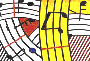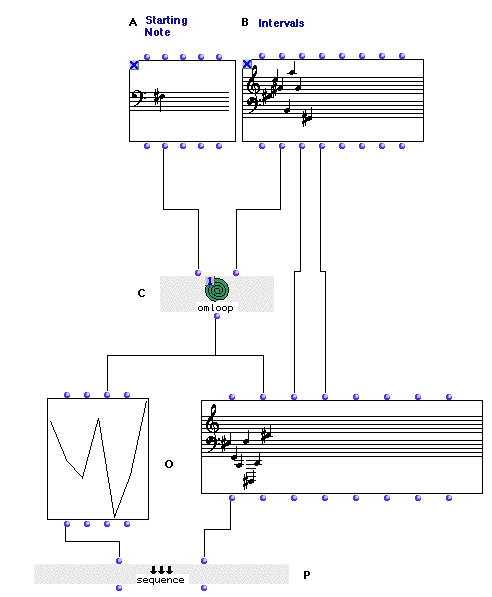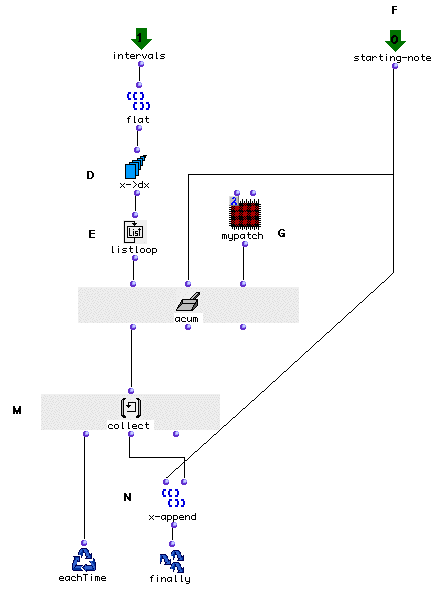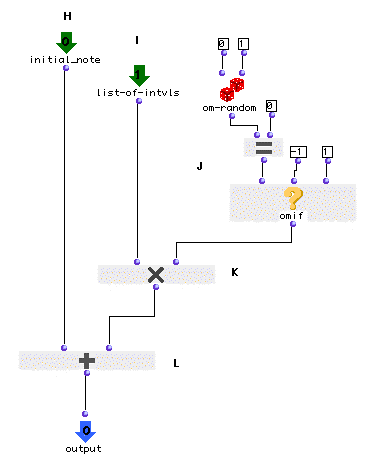Tutorial 37 - Omloop III

Accumulation II

Topics

Constructing a random sequence of notes starting from a given note and agiven note sequence

Functions used

omloop, NOTE, CHORD-SEQ, falt, x->dx, om-random, om=, omif, om* and om+Description

Given a sequence of intervals we will construct a musical sequence starting from a given note. The intervals will be ordered as defined but their direction (up or down) will be random (i.e a minor third per example will be either an ascending or descending one depending on om-random's output). In order to do so we will have to use an accumulative proceedure using omloop's accum collector. We will also visualize our melodic figure using a BPF factory.

Patch structure

A: First we will use te NOTE factory to visualize our starting note.

B: In B we will use a musical figurerepresented in a CHORD-SEQ with determined pitch and rhythm (onsets and durations).

C: The transformation of the sequence into a new one will be processed in the omloop (C). This transformation being only a pitch transformation omloop (C) is connected to the second input of CHORD-SEQ (O) and both the third and fourth outputs of CHORD-SEQ (B) are directly connected to the repective inputs of the new CHORD-SEQ in order to 'copy ' identicaly the initial rhythmic figure.

omloop as shown above is in 'eval-once ' mode. This is due to the fact that omloop is connected to two objects, and we want to be sure that it will be evaluated once for both of these specialy if we are using (as it is the case) random functions. we have already met this case in tutorial_11 ).D: Now let us take a close look inside omloop. First of all we must 'convert' the list of midics we will be receiving through the 'intervals' input into a list of intervals. This is done using x->dx method after using flat which will transform our list of lists (remember this is the output of a CHORD-SEQ) into a simple list.

E: Each interval will be enumerated and 'computed' with the initial starting note (F).

F: The operation is in a form of a lamba patch (G)G: The lambda patch (G) will take the initial note (H) and will add to it the first interval (I). This interval will be first multiplied using om* (K) either by 1 which will leave it as is (i.e if it is a positive integer this will return an ascending interval if it is a negative one it will be a descending interval) or by -1 which will invert the interval.

J: In order to randomly invert the intervals we have used om-random. As we know, om-random returns random numbers between its given limits in input 1 and 2. In our case we had to use a different strategy because we are only interested in having -1 and 1. If we have used priorly om-random with -1 and 1 as arguments it could have returned zeros. So that is where omif comes handy. We used om-random with 0 and 1 as arguments and connected it to the om= predicate in order to transform each zero into a -1. If om-random returns a zero, om= will return 't' and omif in its turn will return -1, else one is returned.

L: Once we have added our first interval (using om+) to the initial note, the result will be the a new note which will be the new state of our accum collector. Again the same process will take place with this new note and will be added to the next interval.

P: In order to have both factories ( the BPF and the CHORD-SEQ) evaluated in a single evaluation we will use sequence. sequence evaluates all its argument sequentially and returns only one in the listener depending on the output evaluated. For instance if we evaluate the first output of sequence we will obtain the BPF object in the listener. Evaluating the second one will return the CHORD-SEQ object.